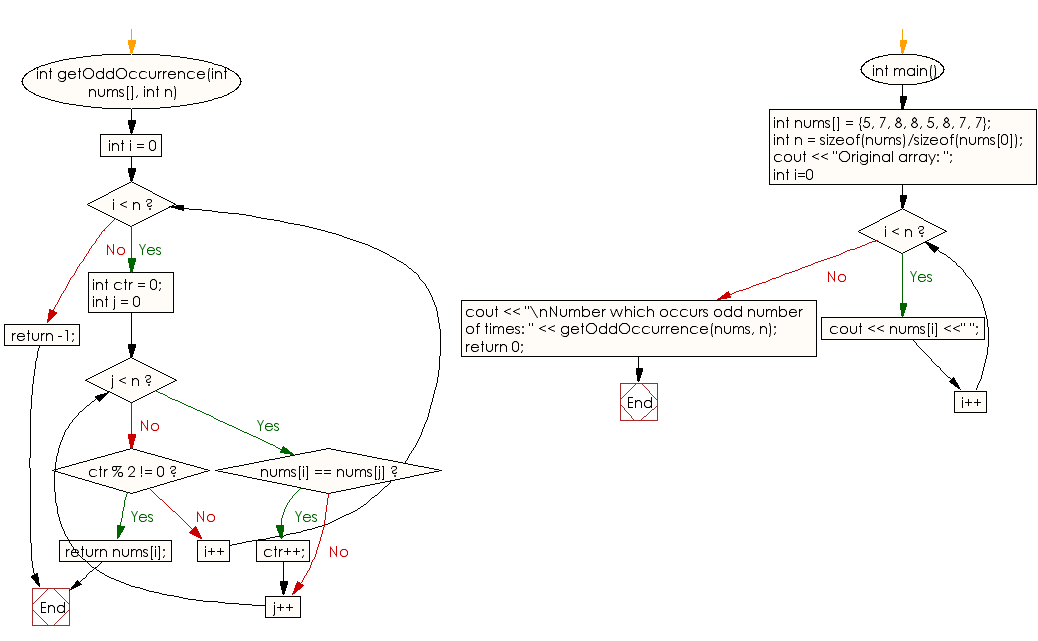﻿ C++ : Find a number occurs odd number of times of an array# C++ Exercises: Find a number which occurs odd number of times of a given array of positive integers

## C++ Array: Exercise-19 with Solution

Write a C++ program to find a number which occurs odd number of times of a given array of positive integers. In the said array all numbers occur even number of times.

Sample Solution:

C++ Code :

``````#include <iostream>
using namespace std;

int getOddOccurrence(int nums[], int n)
{
for (int i = 0; i < n; i++) {

int ctr = 0;

for (int j = 0; j < n; j++)
{
if (nums[i] == nums[j])
ctr++;
}
if (ctr % 2 != 0)
return nums[i];
}
return -1;
}

int main()
{
int nums[] = {5, 7, 8, 8, 5, 8, 7, 7};
int n = sizeof(nums)/sizeof(nums);
cout << "Original array: ";
for (int i=0; i < n; i++)
cout << nums[i] <<" ";
cout << "\nNumber which occurs odd number of times: " << getOddOccurrence(nums, n);

return 0;
}
``````

Sample Output:

```Original array: 5 7 8 8 5 8 7 7
Number which occurs odd number of times: 7
```

Flowchart:C++ Code Editor:

What is the difficulty level of this exercise?

Test your Programming skills with w3resource's quiz.

﻿